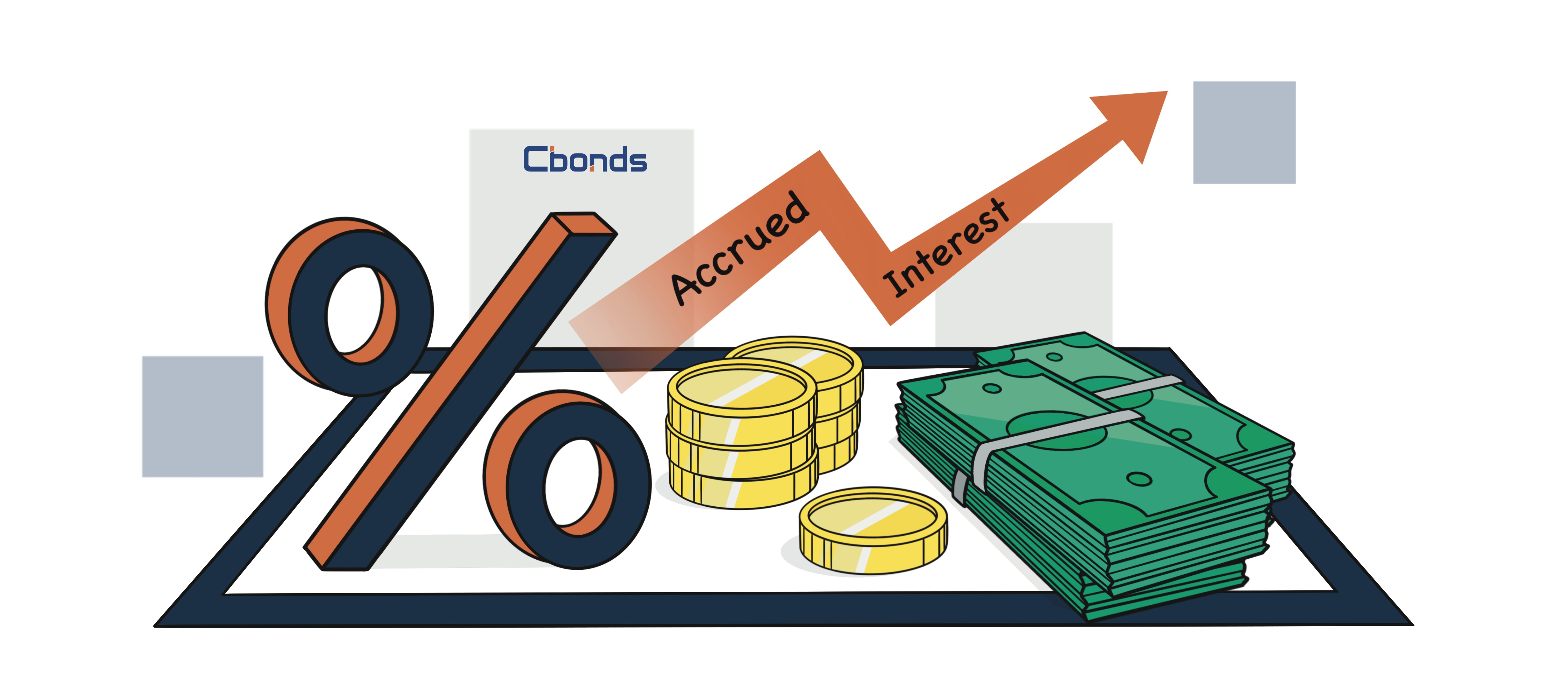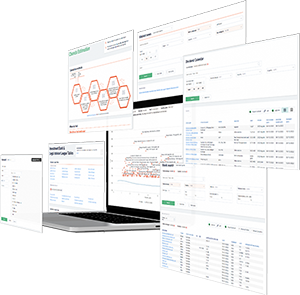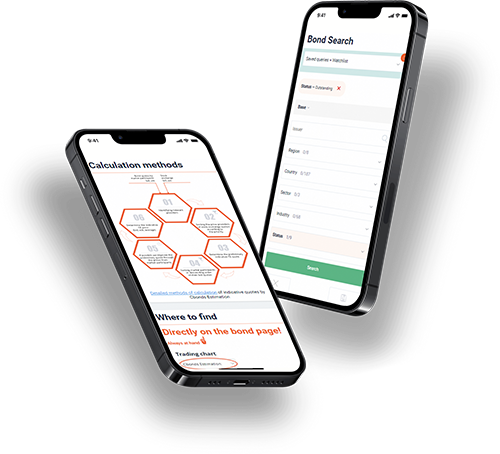Hint mode is switched on
Glossary

# Accrued Interest

Category — Analytical Metrics

## What Is Accrued Interest?

Accrued interest is a vital concept in accounting, denoting the accumulated amount of interest that has been incurred on a loan or any other financial obligation up to a specific date but remains unpaid. It can be categorized as either accrued interest revenue, which applies to the lender, or accrued interest expense, which pertains to the borrower.

Furthermore, accrued interest is also used to describe the sum of bond interest that has accumulated since the previous bond interest payment.

Calculating accrued interest involves considering the interest rate, payment date, and principal balance. The formula for determining accrued interest varies depending on the specific scenario and the terms of the loan or debt.

For instance, if we take an example of accrued interest, let’s assume a loan with an annual interest rate of 5% and a principal balance of \$10,000. To calculate the accrued interest, we need to determine the number of days for which the interest has accrued, the daily interest rate, and the accounting period during which the interest is being calculated.

Accrued interest plays a significant role in financial statements, including the balance sheet and income statement, as it reflects the interest the company owes or has charged its borrowers. It follows accrual accounting principles, where interest accrues over time rather than being paid immediately.## Accrual Accounting and Accrued Interest

Accrued interest represents the outstanding amount of interest that has not been paid yet on a loan or financial obligation. It can be categorized as unpaid interest or accrued interest receivable. For bonds, accrued interest refers to the interest that has accumulated since the last interest payment.

On the other hand, accrued interest payable refers to the amount of interest that a borrower owes but has not yet paid. This is an important consideration when making monthly payments or calculating the amount of accrued interest.

One can utilize tools such as an accrued interest calculator or follow the accrued interest formula specific to the loan or obligation to determine how much accrued interest has accumulated. The amount of accrued interest depends on factors like the interest rate, reporting period, average daily balance, and the next payment date.

Interest payments are the regular payments made to cover the accrued interest. These payments can be on a monthly basis or follow a different schedule depending on the loan terms. When making payments, it is important to record the transaction accurately through a journal entry, following the accrual basis of accounting.

The due date and the timing of payments can vary depending on the loan agreement and the financial institution’s policies. It is essential to be aware of the first interest payment date and any subsequent payment dates to ensure timely payments.

Accrued interest can significantly impact the financial statements of both the borrower and the lender. It affects the net effect on the accounts, and the interest charged or accrued interest payable should be reflected accurately. In some cases, reversing entries may be necessary to adjust for accrued interest.

Accrued interest is an essential aspect of loans and financial obligations, representing the accumulated interest that is yet to be paid. It affects the borrower’s account and the lender’s revenue and requires careful consideration when making payments and recording transactions.

## Accrued Interest in Bonds

Accrued interest plays a crucial role when engaging in the purchase or sale of a bond. Bonds serve as a means of compensating the lender through regular interest payments, commonly known as coupons, typically disbursed twice a year.

If a bond transaction occurs outside the standard interest payment dates, the buyer must account for any accrued interest accumulated since the last interest payment. This accrued interest is added to the sales amount, ensuring the new owner receives a complete semiannual interest payment on the upcoming payment date. Consequently, the previous bondholder is entitled to receive the interest that accrued prior to the sale.

## How to Calculate Accrued Interest

To calculate accrued interest, it’s essential to gather key information beforehand. This information typically includes the interest rate expressed as a percentage, the specific period for which you wish to compute accrued interest, and the loan amount. Here is the formula you can employ with this data to determine the accrued interest:

Accrued interest = [interest rate X (time period ÷ 365)] X loan amount

To ensure proper execution of the formula, follow these order of operations guidelines:

1. Divide the number of days you are considering by the total number of days in a year, which is 365.

2. Multiply the interest rate by the result obtained in step one.

3. Multiply the value obtained in step two by the loan amount.

4. Review and analyze the final result to ascertain the accrued interest amount.

## Accrued Interest Example - Accounting

Let’s consider a scenario where there is a \$20,000 loan receivable with an interest rate of 7.5%. Payment has been received for the period up to the 20th day of the month. To calculate the additional amount of interest revenue earned from the 21st to the 30th of the month, follow this calculation:

(7.5% x (10 / 365)) x \$20,000 = \$41.10

For the party receiving payment, the accrued interest is recorded as a credit to the interest revenue account and a debit to the interest receivable account. The interest receivable is then included in the balance sheet as a short-term asset, while the same amount is reported as revenue on the income statement.

On the other hand, for the party responsible for making the payment, the accrued interest is recorded as a credit to the accrued liabilities account and a debit to the interest expense account. The accrued liabilities are reported on the balance sheet as short-term liabilities, and the interest expense is reflected on the income statement.

Both cases are treated as reversing entries, meaning they are reversed on the first day of the subsequent month. This ensures that when the cash transaction takes place in the following month, only the portion of revenue or expense earned or incurred in the current period remains in that period.

In the given example, the lending company receives \$123.29 (\$20,000 x 7.5% x (30/365)) on the 20th day of the second month. Out of that amount, \$41.10 relates to the prior month and was adjusted through a journal entry to recognize the revenue in the month it was earned. As the adjusting journal entry reverses in the second month, the net effect is that \$82.19 (\$123.29 - \$41.10) of the payment is recognized in the second month, equivalent to the interest earned over the 20 days in that month.

## Accrued Interest Example - Bonds

Let’s consider the scenario of purchasing a bond with a face value of \$1,000 and a semiannual coupon of 5%. The bond makes interest payments on June 1 and December 1, and you plan to buy it on September 30. The question is: How much accrued interest would you need to pay?

In this example, we will use the 30/360 day-count convention, which assumes that each month has 30 days, commonly used in U.S. corporate and municipal bonds.

Step 1. Calculate the number of days between the last coupon payment date (June 1) and your purchase date (September 30). Based on the 30/360 convention, the number of days is 120.

Step 2. Compute the accrued interest by multiplying the day count by the daily interest rate and the face value of the bond.

Accrued interest = 120 x (5% / 360) * \$1,000 = \$16.67

Step 3. Add the accrued interest to the face value of the bond to determine the purchase price.

Purchase price of the bond = \$1,000 + \$16.67 = \$1,016.67

On the subsequent coupon payment date (December 1), you would receive \$25 in interest. However, since you already paid \$16.67 in accrued interest at the time of purchasing the bond, the net interest received by you is \$8.33 (\$25 - \$16.67). This amount represents the interest you should have received for the 60 days that you held the bond until the next coupon payment (from September 30 to December 1).

Get access
×

## — We have everything you need:full data on over 700 000 bonds, stocks & ETFs; powerful bond screener; over 350 pricing sources among stock exchanges & OTC market; ratings & financial reports; user-friendly interface; available anywhere via Website, Excel Add-in and Mobile app.

×

## WhyYou will have detailed descriptive & pricing data for 650K bonds, 76K stocks, 8K ETFsGet full access to the platform from any device & via Cbonds app